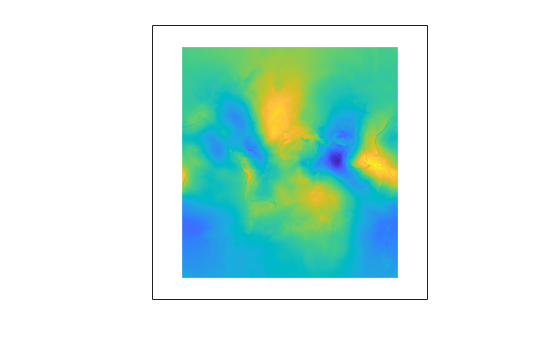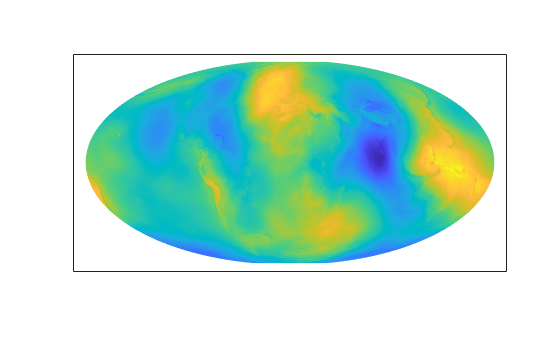## Change Map Projections When Using `geoshow`

You can display latitude-longitude raster and vector data on `axesm`-based maps by using the `geoshow` function. When you change the projection of an `axesm`-based map that contains raster data plotted by the `geoshow` function, MATLAB® reprojects the raster data. However, if the map contains vector data plotted by the `geoshow` function, MATLAB does not reproject the vector data. To reproject vector data, you must remove the vector data from the map and then plot it again.

### Change Map Projections When Using `geoshow`

Change the map projection of an `axesm`-based map after displaying raster and vector data using the `geoshow` function.

Reproject Raster Data

When you change the projection of an `axesm`-based map that contains raster data plotted by the `geoshow` function, MATLAB reprojects the raster data.

Set up an `axesm`-based map that uses a Mercator projection.

```figure axesm mercator```

Display raster data on the map by using the `geoshow` function. For this example, display geoid heights from the EGM96 geoid model.

```[N,R] = egm96geoid; geoshow(N,R,"DisplayType","surface")```Change the map projection to use a Mollweide projection.

```ax = gca; setm(ax,"MapProjection","mollweid")```Reproject Vector Data

When you change the projection of an `axesm`-based map that contains vector data plotted by the `geoshow` function, MATLAB does not reproject the vector data. To reproject the vector data, you must remove the vector data from the map and then plot it again.

Set up an `axesm`-based map that uses a Miller projection.

```figure axesm miller```

Display vector data on the map by using the `geoshow` function. For this example, display a shapefile containing world land areas. Return the plot objects in `v`.

`v = geoshow("landareas.shp");`To reproject the map, first delete the plot objects. Change the map projection to use an orthographic projection. Then, plot the data again.

```delete(v) ax = gca; setm(ax,"MapProjection","ortho") geoshow("landareas.shp")```